# Go Math Grade 3 Answer Key Chapter 6 Understand Division Assessment Test

This chapter can improve student’s math skills, by referring to the Go Math Grade 3 Answer Key Chapter 6 Understand Division Assessment Test, and with the help of this Go Math Grade 3 Assessment Test Answer Key, students can score good marks in the examination.

Go Math Grade 3 Answer Key Chapter 6 contains all the topics of chapter 6 which helps to test the student’s knowledge. Through this assessment test, students can check their knowledge. This assessment test is also helpful for the teachers to know how much a student understood the topics.

Chapter 6: Understand Division Assessment Test

### Test – Page 1 – Page No. 61

Question 1.
For numbers 1a–1d, select True or False for each equation.
a. 5 ÷ 1 = 5
i. True
ii. False

Explanation: 5 ÷ 1 = 5, so answer is true.

Question 1.
b. 3 ÷ 3 = 1
i. True
ii. False

Explanation: 3 ÷ 3 = 1, so the answer is true.

Question 1.
c. 4 ÷ 1 = 1
i. True
ii. False

Explanation: 4 ÷ 1 = 4, not 1. So the answer is False.

Question 1.
d. 0 ÷ 1 = 0
i. True
ii. False

Explanation: 0 ÷ 1 = 0, so the answer is true.

Question 2.
Elian is making 36 ounces of punch. He pours the same amount into each of 6 cups. How many ounces of punch does he pour into each cup?
Circle the amount to complete the sentence.
Elian pours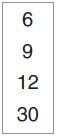ounces of punch into each cup.
_____

Explanation:

Elian poursounces of punch into each cup.

Question 3.
Gina arranges her 21 puzzles in 7 equal stacks. How many puzzles does Gina put in each stack?
Shade squares to make an array to model the problem.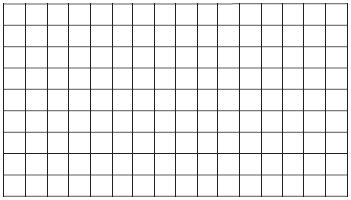Explanation: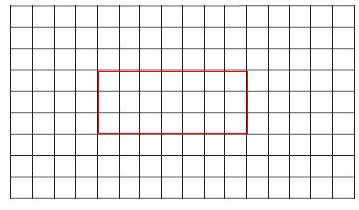### Test – Page 2 – Page No. 62

Question 4.
Philip has 30 pennies that he exchanges for nickels. He exchanges 5 pennies for each nickel. How many nickels does Philip get?
Make equal groups to model the problem._____ nickels

Explanation: As Philips has 30 pennies and he exchanges 5 pennies for each nickel, so Philips get 30÷5= 6 nickels.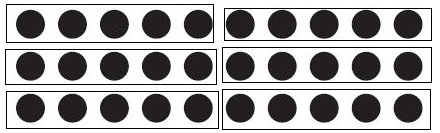Question 5.
A book has 54 pages divided into 6 equal chapters.
How many pages are in each chapter?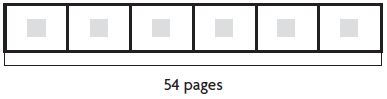_____ pages

Explanation: 54÷6= 9 pages.

Question 6.
Select the equations that the array represents.
Mark all that apply.Options:
a. 2 × 4 = 8
b. 3 × 2 = 6
c. 6 ÷ 3 = 2
d. 2 × 3 = 6
e. 6 ÷ 2 = 3
f. 8 ÷ 2 = 4

Explanation:
3 × 2 = 6
6 ÷ 3 = 2
2 × 3 = 6
6 ÷ 2 = 3

### Test – Page 3 – Page No. 63

Question 7.
The 16 players at tennis camp are divided into 4 equal groups. How many players are in each group?
Part A
Draw jumps on the number line to model the problem.Type below:
___________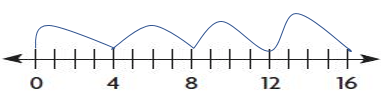Question 7.
Part B
Write a division equation to represent the model.
_____ ÷ _____ = _____

Explanation: 16÷4= 4

Question 8.
Max writes a total of 45 lines in his journal. Each journal entry is 9 lines long. How many journal entries does Max make?
Choose a number from the box to complete the sentence.Max makes _____ journal entries.
_____

Explanation: Max writes a total of 45 lines in his journal and each journal entry is 9 lines long, so 45÷9= 5 journal entries does Max makes.

Question 9.
A farmer sells baskets that hold 6 peaches each. Etta buys a total of 18 peaches. How many baskets of peaches does Etta buy?
Complete each equation to represent the problem.
6 × _____ = 18;    18 ÷ 6 = _____ ;     _____ baskets

Explanation: As the farmer sells baskets that hold 6 peaches each and Etta buys a total of 18 peaches, the number of baskets of peaches does Etty bought is 18÷6= 3 baskets.
6 × 3 = 18;
18 ÷ 6 = 3

Question 10.
Penn has 12 eggs to use in some recipes. Select a way that he could divide all of the eggs equally among the recipes. Mark all that apply.
Options:
a. 6 eggs in each of 2 recipes
b. 5 eggs in each of 3 recipes
c. 3 eggs in each of 4 recipes
d. 4 eggs in each of 4 recipes
e. 2 eggs in each of 6 recipes
f. 4 eggs in each of 3 recipes

Explanation:
6×2= 12
3×4= 12
2×6= 12
4×3= 12

### Test – Page 4 – Page No. 64

Question 11.
Nancy made this array to model a division equation. Which equation could Nancy have modeled? Mark all that apply.Options:
a. 63 ÷ 7 = 9
b. 54 ÷ 6 = 9
c. 72 ÷ 9 = 8
d. 63 ÷ 9 = 7

Explanation:
63 ÷ 7 = 9
63 ÷ 9 = 7

Question 12.
Rico went for a bike ride around a 3-mile loop. He rode a total of 12 miles. How many times did Rico ride his bike around the loop?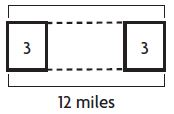_____ times

Explanation: As Rico went for a bike ride around a 3-mile loop and rode a total of 12 miles, so 12÷3= 4 times Rico ride his bike around the loop.

Question 13.
Write the letter for each problem in the box next to its quotient.
Options:
A. 5 ÷ 5 _____ 0
B. 0 ÷ 5 _____ 5
C. 5 ÷ 1 _____ 1

5 ÷ 5= 1
0 ÷ 5= 0
5 ÷ 1= 5.

Question 14.
Karson earns $6 each hour babysitting. Last week she earned a total of$48 babysitting. How many hours did Karson babysit last week?
Choose a number from the box.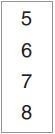______ hours

Explanation: As Karson earns $6 each hour babysitting and in last week she earned a total of$48 babysitting, so $48÷$6= 8 hours did Karson earn in babysit last week.

### Test – Page 5 – Page No. 65

Question 15.
Write the division equation to represent the repeated subtraction._____ ÷ _____ = _____

Explanation:
15÷5= 3

Question 16.
Write the related facts for the array.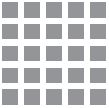5×5= 25
25÷5= 5

Explain why there are not more related facts for the array.

Answer: There are only two equations, as both factors are the same.

Question 17.
Sierra separates 14 animal cards into equal piles with none left over.
Draw a picture to show how Sierra divides the cards and complete the sentence.
Sierra made ______ piles of cards.

Explanation: Here we must know how 14 can be divided. So 14 can be divided into two ways, 7×2= 14 and 2×7= 14. So Sierra made 2 piles of cards.

Could Sierra have separated the cards into 4 equal piles with none left over? Explain why or why not.

Explanation: Sierra cannot separate into 4 equal piles, as 14 cannot be divided into 4 groups of equal size.

### Test – Page 6 – Page No. 66

Question 18.
Circle numbers to complete the related facts.× 6 = 42 42 ÷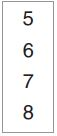= 6
_____ _____

Explanation:
7×6= 42
42÷7= 6

Question 19.
Use numbers from the box to write related multiplication and division facts.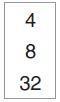_____ × _____ = _____
_____ × _____ = _____
_____ ÷ _____ = _____
_____ ÷ _____ = _____

8×4= 32
4×8= 32
32÷4= 8
32÷8= 4

Question 20.
Angela plants 24 rosebushes in flowerbeds in her yard. She plants the same number of rosebushes in each of 6 flowerbeds.
How many rosebushes does Angela plant in each flowerbed? Show your work.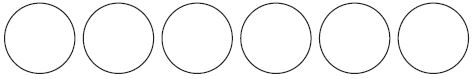______ rosebushes